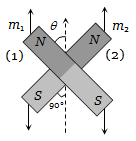NEET Physics Magnetism and Matter Questions Solved

Two magnets of equal mass are joined at right angles to each other as shown the magnet 1 has a magnetic moment 3 times that of magnet 2. This arrangement is pivoted so that it is free to rotate in the horizontal plane. In equilibrium what angle will the magnet 1 subtend with the magnetic meridian(a) ${\mathrm{tan}}^{-1}\left(\frac{1}{2}\right)$

(b) ${\mathrm{tan}}^{-1}\left(\frac{1}{3}\right)$

(c) ${\mathrm{tan}}^{-1}\left(1\right)$

(d) $0°$

Concept Videos :-

#10 | Earth's Magnetism
#11 | Apparent & True Dip
#12 | Neutral points
#13 | Comparison of Magnetic Field of Earth at Two Points
#14 | Variation of Earth's Magnetic Field

Concept Questions :-

Earth's magnetism
Explanation is a part of a Paid Course. To view Explanation Please buy the course.

Difficulty Level: